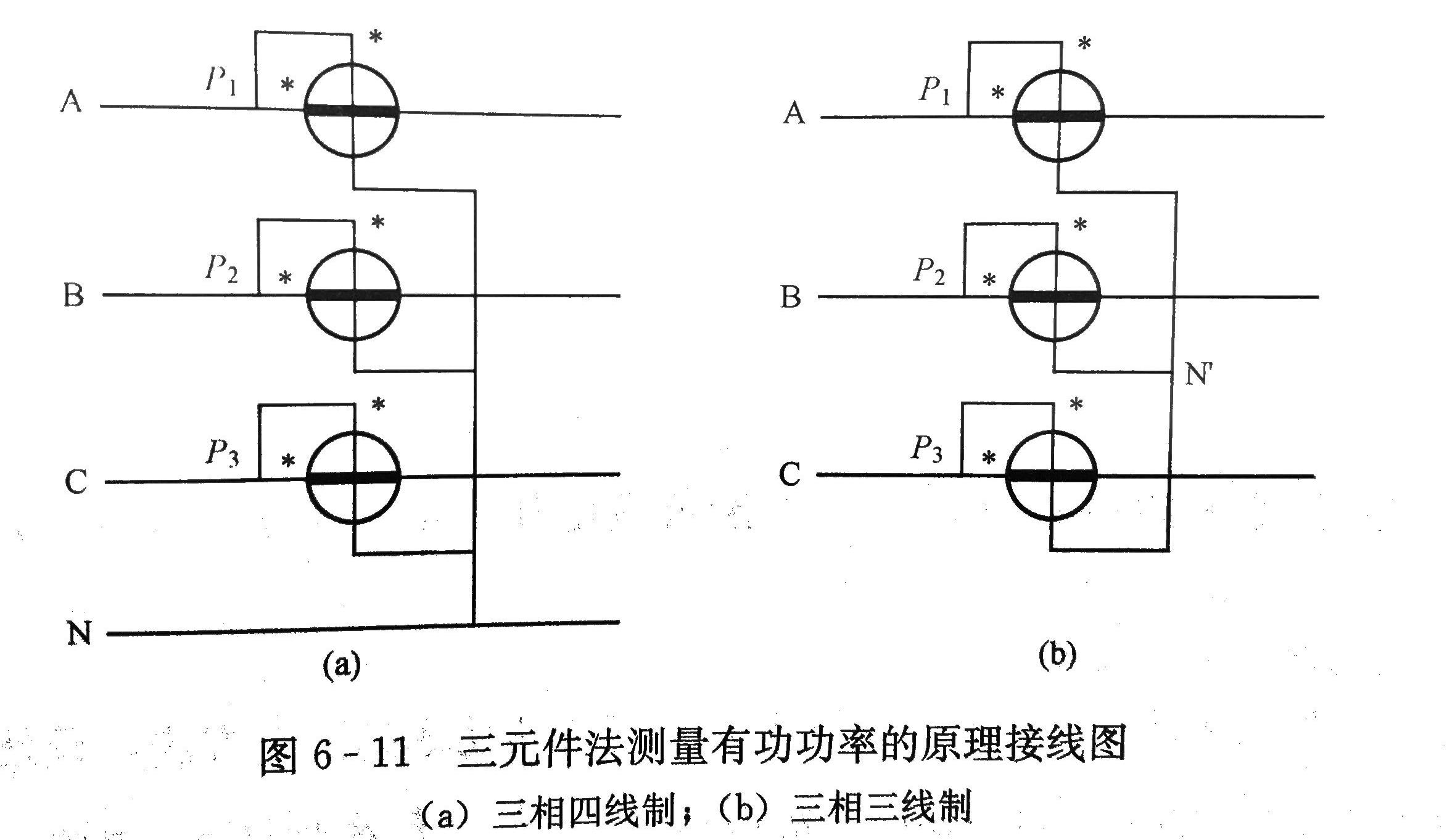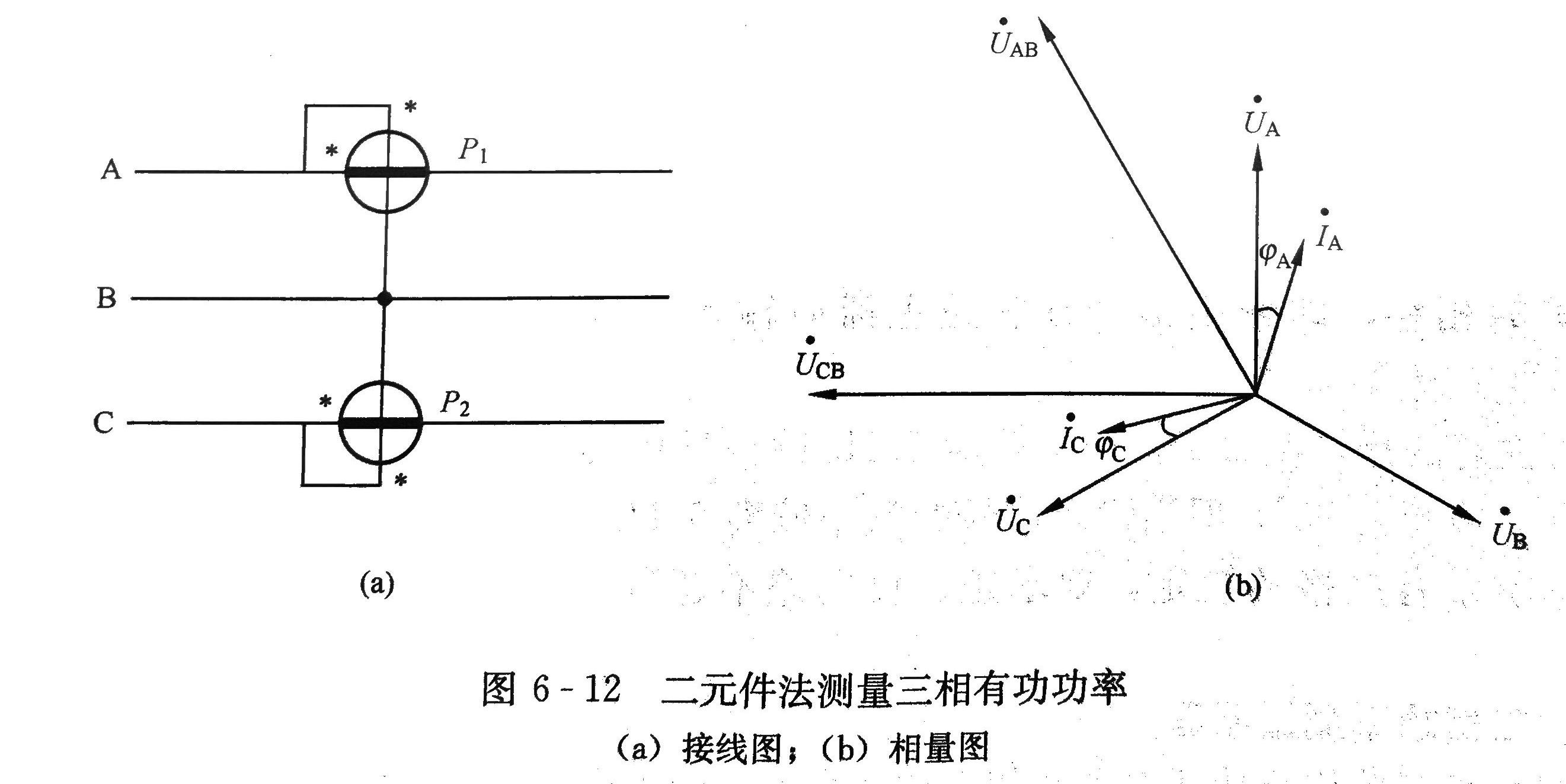• 单选题 20分
• 判断题 20分
• 简答题 5*6=30分
• 综合题 30分
• 考试范围：第一章~第七章

## 第一章

### 远动信息的编码

CDT模式：

• 8位功能码：区分代表不同信息内容的各种信息字
• 32位信息码：信息内容
• 8位校验码：用于检错和纠错，提高信息字在传输过程中抗信道干扰的能力，采用CRC校验

polling模式：

• 问答双方RTU地址
• 报文类型
• 报文数据区字节数
• 信息内容
• 校验码

• 专用有线信道
• 复用电力线载波信道
• 微波信道
• 光纤信道
• 无线电信道

### 远动系统的构成及各部分功能RTU对各种电量变送器送来的0~5V直流电压分时完成A/D转换，得到与被测量对应的二进制数值；并由脉冲采集电路对脉冲输入进行计数，得到与脉冲量对应的计数值；海拔状态量的输入状态转换成逻辑电平“0”或“1”。再将上述各种数字信息按规约编码或遥测信息字和遥信信息字，向前置机传送。

## 第二章

### 数字通信系统模型• 信息源：将消息转换成信号输出
• 信源编码：对信息源发出的模拟信号完成A/D转换，得到对应的数字信号。然后对数字信号进行编码得到一串离散的数字信息m。
• 信道编码/差错控制编码：按照一定的规则，在信息序列m中添加一些冗余码元，将信息序列m变成较原来更长的二进制数字序列c，称为码字，提高信息序列m的抗干扰能力。
• 调制：将用数字序列表示的码字c变换成适合于在信道中传输的信号形式，送入信道。
• 信道：传输信号的通道
• 解调：把从信道中接收到的两种不同频率或两种不同相位的正弦交流信号，还原成数字序列。
• 信道译码：根据信道编码规则，对接收码字进行译码校验，达到检出或纠正接收码字R中错误码元的目的，并产生出估计与发送码字c对应的接收码字c*，再从c*还原出估计与信息序列m对应的m*
• 信源译码：根据信源编码规则，变换信息序列m*为信息源输出s的对应值s*，并送给收信者予以显示或打印等。

### 通信方式

• 单工通信：信息的传输始终是一个方向，不能进行与此相反方向的传输。
• 半双工通信：信息可以在两个方向上传输，但某一时刻只限于一个方向，不能同时进行双向传输。
• 全双工通信：信息可以同时做双向传输。

### 异步通信与同步通信

• 异步通信：按字符传送，每个字符以起始位开始，以停止位结束。每个字符的起始位都起到对该字符的位同步作用，使频率的漂移不会积累。异步通信时，收发双方必须设置相同的字符格式。
• 同步通信：以同步字符作为传送开始，字符与字符之间不允许有空隙，当线路空闲或没有字符可发时，发送同步字符。同步通信时，收发双方必须设置相同的同步字符，另外收发双方必须保证有同步的时钟，由他们分别控制信号的发送与接收。

### CDT传输规约帧结构### CDT传输规约信息字结构### 帧的组织方式

• 重要遥测量安排在A帧传送，循环时间不大于3s
• 次要遥测量安排在B帧传送，循环时间不大于6s
• 一般遥测量安排在C帧传送，循环时间不大于20s
• 遥信状态信息，包含子站工作状态信息，安排在D1帧定时传送
• 电能脉冲计数值安排在D2帧定时传送
• 事件顺序记录（SoE）安排在E帧，以帧插入方式传送三遍

### 问答式传输规约 主站与子站间的问答过程RTU刚合电源时，子站向主站发送电源合闸报文，主站收到后向子站送电源合闸确认命令，子站收到后对该命令回答确认报文。然后主站通过各种送参数命令对RTU的各种参数初始化。最后反复用类别询问获取RTU的实时数据，直至RTU的所有量送完为止，以便主站建立数据库。

## 第三章

### 最小距离与码的检错、纠错能力

\begin{aligned} t&\le\cfrac{d_{min}-1}{2}\\ l&\le d_{min}-1 \end{aligned}

### 模2运算

#### 加法运算规则

$$\begin{gather} 1\oplus1=0\\ 0\oplus0=0\\ 0\oplus1=1\\ 1\oplus0=1 \end{gather}$$

#### 乘法运算规则

$$\begin{gather} 1\odot1=1\\ 0\odot0=0\\ 0\odot1=0\\ 1\odot0=0 \end{gather}$$

$$m(x)=m_{k-1}x^{k-1}+m_{k-2}x^{k-2}+\cdots+m_1x+m_0$$

$$c(x)=c_{n-1}x^{n-1}+c_{n-2}x^{n-2}+\cdots+c_1x+c_0$$

### 线性分组码生成矩阵

$$r_2=m_2+m_0\\ r_1=m_2+m_1\\ r_0=m_1+m_0$$

$$c=c_5c_4c_3c_2c_1c_0=m_2m_1m_0r_2r_1r_0$$

$$[c_5c_4c_3c_2c_1c_0]=[m_2m_1m_0] \begin{bmatrix} 1&0&0&1&1&0\\ 0&1&0&0&1&1\\ 0&0&1&1&0&1 \end{bmatrix} =[m_2m_1m_0]G$$

### 循环码的编译码原理

$(n,k)$次循环码的生成多项式$g(x)$是$x^n+1$的一个因式：

$$x^n+1=g(x)h(x)$$

1. 将$x^n+1$进行因式分解，找出一个次数为$n-k$的因式
2. 以这个因式位生成多项式，分别乘以$2^k$个不同的信息序列，得到$2^k$个码字

$(n,k)$系统循环码的编码过程是：

1. 将信息多项式$m(x)$乘以$x^{n-k}$得到$x^{n-k}m(x)$
2. 以生成多项式$g(x)$除$x^{n-k}m(x)$，若商为$q(x)$，余式为$r(x)$，则$x^{n-k}m(x)=q(x)g(x)+r(x)$
3. 用$r(x)$模2加$x^{m-k}m(x)$，得到系统循环码码字$x^{m-k}m(x)+r(x)$

### 循环码的编译码算法——软件表算法Ⅰ

1. 把信息序列分成长度为$n-k$的$p$个信息段，记为$m=M_1M_2\cdots M_p$
2. 在第一个信息段$M_1$后面添加$n-k$个零，然后除以生成多项式$g(x)$得余数$r_1$。再将余数与第二个信息段$M_2$模2加得$M'_2$
3. 在$M'_2$后面后面添加$n-k$个零，然后除以生成多项式$g(x)$得余数$r_2$，将余数与第三个信息段$M_3$模2加得$M'_3$
4. 重复步骤，直到对$M'_p$按同样步骤得到$r_p$，它就是信息序列$m$编码应得的余数。这时信息序列$m$对应的码字为$c=M_1M_2\cdots M_pr_p$

### 循环码的编译码算法——软件表算法Ⅱ

1. 把信息序列分成长度为$(n-k)/2$的$p$个信息段，记为$m=M_1M_2\cdots M_p$
2. 在第一个信息段$M_1$后面添加$n-k$个零，然后除以生成多项式$g(x)$得中间余数$r_1$。再将$r_1$分成两段，前半段$(n-k)/2$位是高位段，记为$r_{1H}$，后半段是低位段，记为$r_{1L}$
3. 将$r_{1L}$与第二个信息段$M_2$模2加，得$M'_2$。在$M'_2$后面添加$n-k$个零，然后除以生成多项式$g(x)$得中间余数$r_2$。再将$r_2$分成两段，前半段$(n-k)/2$位记为$r_{2H}$，后半段记为$r_{2L}$。
4. 将$r_{2H},r_{1L}$与第三个信息段$M_3$模2加，得$M'_3=r_{2H}+r_{1L}+M_3$。在$M'_3$后面添加$n-k$个零，然后除以生成多项式$g(x)$得中间余数$r_3$。再将$r_3$分成两段，前半段$(n-k)/2$位记为$r_{3H}$，后半段记为$r_{3L}$。
5. 重复步骤，直到求出$M'_p=r_{(p-1)H}+r_{(p-2)L}+M_p$.在$M'_p$后面添加$n-k$个零，然后除以生成多项式$g(x)$得中间余数$r_p$。再将$r_p$分成两段，前半段$(n-k)/2$位记为$r_{pH}$，后半段记为$r_{pL}$。
6. 将$r_{pH}$与$r_{(p-1)L}$模2加得$r_H=r_{pH}+r_{(p-1)L}$，令$r_L=r_{pL}$,则$r_H,r_L$分别是信息序列$m$的余式$r$的高$(n-k)/2$位和低$(n-k)/2$位，$r=r_Hr_L$

## 第四章

### 同步字的检测## 第五章

### 定时扫查模式### 变位触发模式### 事件顺序记录、建立全网统一时钟的方法

#### 统一全网时钟的方法

1. 主站向RTU发送设置时钟命令
2. RTU接收后修改RTU的实时时钟
3. 主站再向RTU发送召唤子站时钟命令
4. RTU收到后插入返送两个信息字，即RTU时钟和等待时间
5. 主站收到返送信息后，计算出RTU的时间校正值，向RTU发出设置时钟校正值命令
6. RTU收到校正值后修正RTU的实时时钟

### 遥测量的转换过程• 一阶递归滤波
• 限幅滤波
• 算术平均滤波
• 递推平均滤波
• 中位值滤波

### 远动规约中的遥控命令

#### CDT模式

1. 主机（调度端）向子站（RTU）发送遥控选择命令
2. 子站向主站返送遥控返较信息
3. 根据实现情况，主站向子站下达遥控执行命令或遥控撤销命令
4. 如果遥控执行则RTU改变遥控输出状态，如果是遥控撤销则遥控状态保持不变

#### 问答式

1. 主站向RTU发送一个遥控对象、性质的选择命令报文
2. 由RTU向主站返送一个返送校核码的报文
3. 主站向RTU发送一个执行命令
4. RTU向主站报告一个正确接收的确认信号
5. 主站向RTU发送询问报文

### 遥调的两种实现方式

• 模拟整定值调节方式
• 正增值/负增值脉冲调节方式

## 第六章

### 交流电流/电压变送器原理图### 功率变送器的原理框图### 三相功率测量原理

#### 三相有功功率的测量#### 三相无功功率的测量### 交流采样算法

• 时域分析算法

• 积分算法
• 二点算法
• 频域分析算法

• 离散傅里叶变换
• 快速傅里叶变换## 第七章

### 二进制数字调制

$$u(t)=U_m\cos(\omega t+\varphi)$$u(t)= \left\{ \begin{align} &0&"0"\\ &U_m\cos\omega t&"1" \end{align} \right.

#### 移频键控（FSK）

u(t)= \left\{ \begin{align} &U_m\cos2\pi f_1t=U_m\cos\omega_1 t&"0"\\ &U_m\cos2\pi f_2t=U_m\cos\omega_2 t&"1" \end{align} \right.

#### 移相键控（PSK）

u(t)= \left\{ \begin{align} &U_m\cos(\omega t+0)&"0"\\ &U_m\cos(\omega t+\pi)&"1" \end{align} \right.

### FSK信号的解调### PSK信号的解调